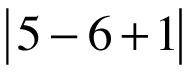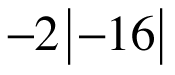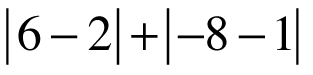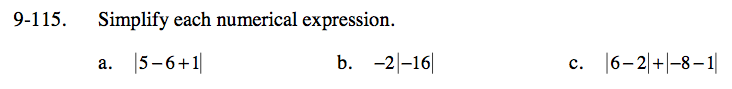Home > CC3 > Chapter 9 > Lesson 9.2.4 > Problem9-115

9-115.
1. Simplify each numerical expression. Homework Help ✎

1.2.3.Simplify within the absolute value signs.

What is 5 − 6 + 1?

|5 − 6 + 1| = |0|

The absolute value of 0 is 0.

First find the absolute value of −16.

The absolute value is the distance a number is away from 0, and is always a non negative number. Since −16 is 16 units away from zero, the absolute value is 16.

What is the product of -2(16)?

Simplify within the absolute value signs first.

6 − 2 = 4 and −8 − 1 = −9. Therefore we have:
|4| + |−9|

What is the absolute value for each of these numbers?

4 + 9 = 13